# Gentle Introduction to Realtime Fluid Simulation for Programmers and Technical Artists

A simple intuitive breakdown of fluid simulation for programmers and technical artists. Instead of complicated mathematical expressions, I try my best to offer an easy geometrical understanding of fluid simulation. The implementation is done in Compute shaders using Unity 3D, however the ideas are technology independent.

As usual, you can find the code for the project on my Github: https://github.com/IRCSS/Compute-Shaders-Fluid-Dynamic-

The resources on fluid simulations can be very daunting. I remember the first time I read a paper on it and saw the Navier Stoke equations, I got very intimated. Over time, I realized the topic itself is not complicated at all. As a matter of fact, if you were given the task of writing a fluid simulator of your own, you will probably end up with making something similar just based on your intuitive understanding of how fluids work. If you look at the Stoke Navier equations and think, “ah, I see, that makes sense”, then you will probably have a faster time implementing a fluid simulation, by reading the papers cited in resources at the end of this article. Here, I try to stay away from condensed expression, and explain things as slowly as I can.

Quick summary of the post. At the beginning, we will ignore the existing Stoke Navier equation, and try to come up with our own implementation of fluid simulation. Keep in mind, that this first implementation will be a naïve implementation that is flawed. We will do it anyway, so that once we add complexity to our implementation to counter these flaws, you will understand why they are there, and can peek through the complexity and see the core implementation. Also keep in mind that a lot of the explanations I provide here have the purpose of explaining the phenomena in simple terms, and offer you an intuitive understanding. These formulations are neither the most accurate nor the most effective way of describing the different elements, but are more than enough for programming a believable effect.

## Properties of Fluids

First lets observe references from the real world. Pour yourself a glass of water, play around with it, and see what visual characteristics of water you have to imitate, for it to be perceived as a believable body of water. Pour a drop of soya sauce in there, and observe how it moves around as you move your finger through the water, or keep it still.

You might notice the following three points:

First, if you pour a drop of ink or soya sauce in your glass, even if you don’t shake the glass or stir the water, the soya sauce will naturally spread around and diffuses until it is uniformly distributed in your water.

Second, you can stir the water. If you put your finger in the glass and moved it around, you will create a series of motions/ velocities, which will continue to exist and effect each other, long after you have removed your finger from the water. So the force, you apply to the body of water, creates some motion in the water. A person can add things to this system, like force or ink. Also notice how the velocities also seem to diffuse after a while and spread around.

Third, based on the direction of the force you apply to the surface, the dye, ink or soya sauce, will be carried around by the body of water. So the body of water has velocities, and the substances inside it are transferred/ advected, by the motion of the water molecules in that area.

Take a break here, and think about how you could code the above elements. Keep in mind, that to any given time, different sections in your glass of water, have different density of soya sauce, and different velocities which change over time.

There are two ways that people usually use to solve problems like these. Number one is to simulate the phenomena through particles that represent a collection of water molecules. Number two is a simulation grid, where each cell represents the state of that section of the body of water. The state of that cell consists of different fields, such as velocity, temperature, dye quantity etc., which change with regards to the motion in the system. You can of course have a combination of both methods. In this post, I am using a grid.

Also, I am going to model everything in 2D, since it is simpler for explaining. Once you understand the core idea, expanding it to 3D is not difficult.

We have a 2D grid, where each cell represents the state of the fluid for that section. The cells are spatially bound, meaning they are referring only to that section of the water and are not bound to the actual water molecules. The molecules will move through these cells, and carry with themselves quantities such as dye, or their own velocity. Our goal is to calculate the state of the fluid, for each of these cells, every frame. So in each frame, the simulation advances forward, based on the result of the previous frame and whatever happened in that frame.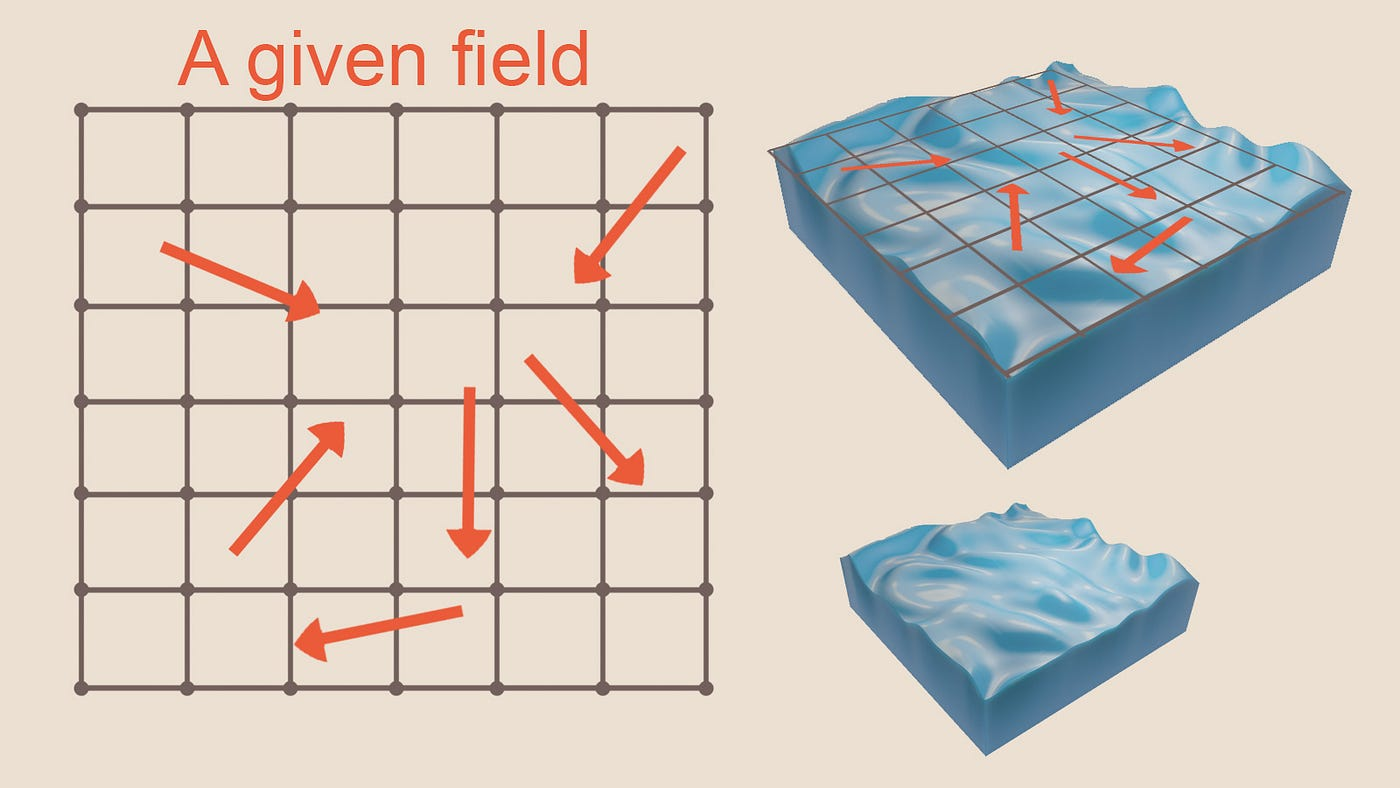Figure -1- Defining a body of water as series of fields on a cell

Based on our three observations, you will find the quantity of the field you are interested in for each cell, based on these three factors:

`AmountOfSomethingInThisCell = What was spread from neighbouring cells + what was carried over from elsewhere due to the motion of the water + what was added in that frame by the user to that cell`

In other words

`FluidStateForFieldX = Diffusion_of_X + Advection_of_X + X_Added_by_the_user`

All we have to do is program each of these different factors every frame, and apply them to every field in our fluid, updating the fields for the next frame and rendering the results.

## Naïve Implementation, Diffusion

As stated before, dropped soya sauce diffuses and spreads around the point where it was added to the body of water. If you think about it, this is a simple exchange process. Each frame for any given cell the dye bleeds to the neighboring cells and the dye of the neighboring cell bleed into that given cell. Over time, the dye will bleed everywhere on your grid, and the entire grid will reach a uniform value for the amount of dye it holds, just like our reference video.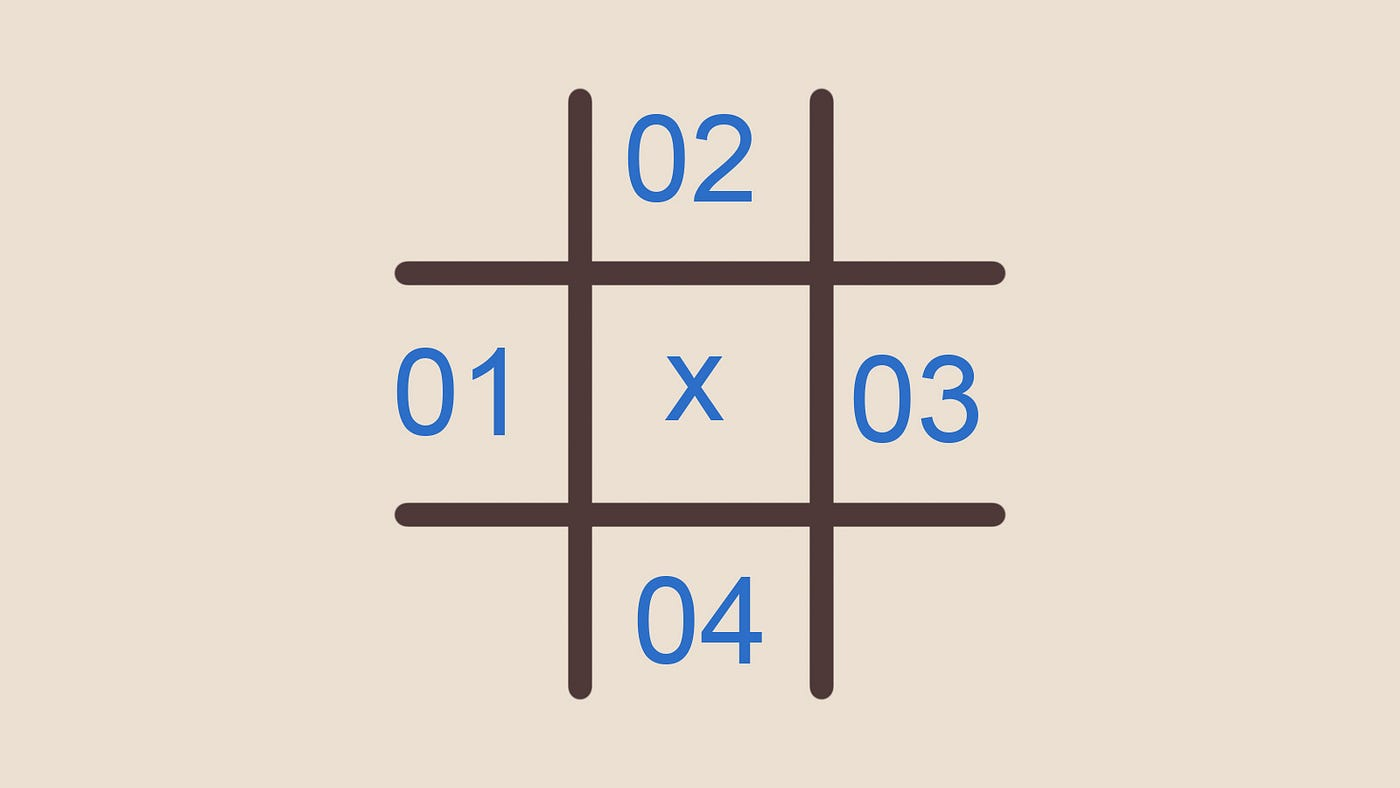Figure -2- Grid A, field marked x is the cell currently being processed

So given the grid (A) let the amount of dye of this frame be d and amount of dye previous frame d0, to calculate the amount of dye on any given cell for the current frame (d) is:

`d_X= d0_X + diffusionFactor * deltaTime (d0_01 + d0_02+ d0_03 + d0_04 -4*d0_X)`

The logic behind this is that every cell gives out 4 portions of the dye it has, and receives one portion from every neighboring cells, or an equal statement would be for every quarter portion of dye the cell receives, it gives an entire one out. Remember that the diffusion factor (a constant set by us) and delta time make the diffusion part rather small, so the cell doesn't actually give out 4 times its own dye amount (that would be physically impossible) and the numbers are just ratios.

So far so good. This is simple enough to implement on the GPU or CPU.

Advection can also be implemented very simply. Each frame, you need to read the amount of velocity of the cell you are assessing, and consider that the molecules in that cell, are going to move with that speed in the direction of the velocity, and carry any substance in the water with them. So, we can read that velocity, read the density of the field we are interested in for that cell, and move that across the grid with that velocity to wherever it would have landed, given the delta time between this frame and the next time we will assess the field, so the next frame. This method projects the field into the future state, using the velocity.

`Field_amount_for_target[cellPosition + current_cell_velocity * timestep] = field_amount_for_current_cell`

## Implementation, User Input

This is the only part where our first implementation will be our final one. Just calculate the user input amount for each cell you are assessing, and add it to the existing value of the field for that cell. How you calculate this can be very different, for example, through mouse input, through a constant stream of input, a moving one, noise, an image etc.

`Amount_of_field_X += user_input_for_this_cell`

If you implement the above, you might find something that resembles fluid behavior (for example the diffuse part will make it look like watercolor hitting paper), and you could even implement it in the CPU without much problem as far as things remain single threaded. However, the current implementation has three major flaws: the fluid is compressible, it is not suitable for multithreading and it is unstable for larger timesteps.

## Flaw One, Compressible Fluids?

One of the key characteristics of fluids is those beautiful curls that pop up as you move your finger through the water. The question is, why do these curls pop up, and why doesn't our simulation have it?

Consider the following scenario. In the grid below, all neighboring grids have velocities that point towards a single cell. If all these molecules are being carried over the next frame to this cell, how are they going to fit in there? Are they going to get compressed?

Or this other scenario, the velocities are constantly pointing away from the central cell. Overtime, where would these molecules come from, is matter being created out of nothing?

The above scenarios elude to something our simulation is lacking, which is fluids can not be compressed (or at least that is an assumption good enough to get a believable fluid simulation going). Nowhere in our current simulation are we compensating for the fact that we can’t endlessly push water to a segment of space without any consequences.

The incompressibility of fluids is the reason why those beautiful curls pop up in fluid bodies. Intuitively, you can think about it like this, if you are moving something forward, and it cant possibly be push forward, then if it can, it will move to the sides, which creates the curls. We fix this through projection which we will cover right after going over the other two flows.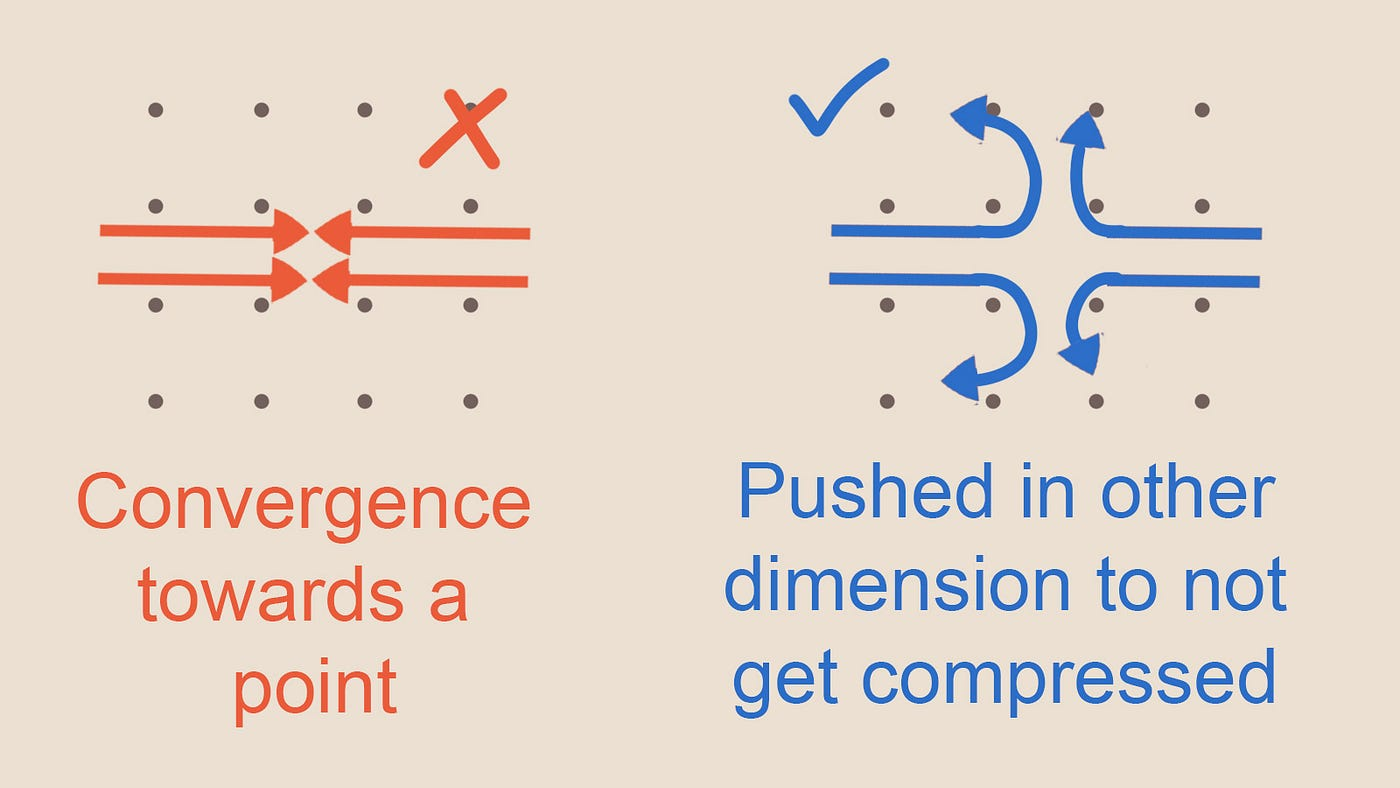Figure -6- fluid moving to where it can because it cant get compressed

## Flaw Two: Bad For GPU Implementation

Lets look at our advection calculation again. Each cell looks at the velocity it currently has, then carries whatever field quantity it has based on that velocity, to wherever the velocity would reach given the time step. In other words, each cell is not writing to its own memory, but some unknown location. This type of access pattern is called a scatter operation.

If we think about multithreading (CPU or GPU), we have a problem. If we map each thread to a cell, threads would be writing to memory locations, where potentially other threads want to also write to. This creates a racing condition.

Ideally we want a setup where a thread is mapped to a cell and each thread only writes to the memory of the cell it is assigned to, while only reading from the neighboring cells. This would be an example of a gather operation. GPUs are great for gather operations.

## Flaw Three: Instability

This is a hard one to explain and is beyond the scope of this article in its full glory.

In the way we are calculating the advection and diffusion right now, we define the value of the velocity in the future, based on its value in the past (previous frame), the time step (frame delta time) that has passed between the past and the present and how this value was changing in the previous frame (defined based on some function we derived from our reference and deduction). The value at any given time is explicitly defined through these factors .

The issue with this is that if this delta time or in case of diffusion the delta time and/or diffusion factor get large (larger than the size of our cells in advection or the amount of dye in the cell in diffusion for example), due to some of the terms in our advection/ diffusion formulation, the solution will become unstable and oscillate (switch between a positive and negative, or a smaller and larger answer every frame) and eventually blow up to very large numbers. So stability here is not about accuracy of the result, but rather whether the result will converge to a stable value over time.

Let’s build a simple theoretical case. We are iteratively advancing the state of our simulation, by updating the values each frame. This is at best an estimation. Imagine you wanted to reproduce the function (A) iteratively, at any given time you take your pre-existing value of the function at the frame before (which you know) and you add the slope of the function at that point to advance your estimation of the function forward. This slope is however an instantaneous slope, and for large jumps in time, it will completely over shoot the function, as illustrated below. Notice how even with small delta time, the blue points (our approximate solution to the problem) don’t lie exactly on the red line. There is always some error to our approximation.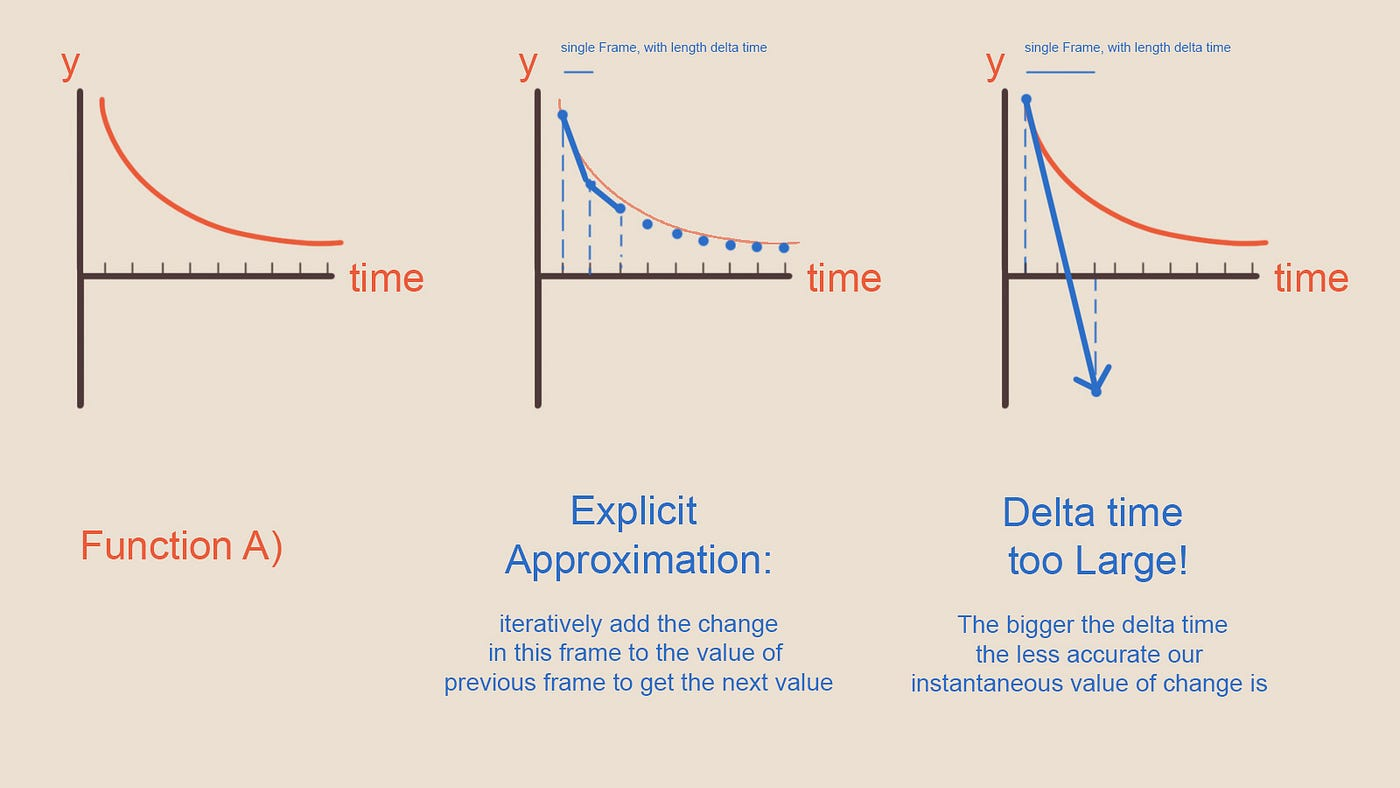Figure -7- Too large delta time lead to values blowing up. Each step has a local error which compounds over time to very large values

I won’t go further into details, because we will automatically get rid of this problem in advection as we switch from a scatter operation to a gather operation and we rephrase our diffusion calculation to get rid of this problem. In the new setup for diffusion the value of the cells are implicitly defined by their dependency to the value of the field for every other cell. This method is stable for a larger time step, though the values might still be inaccurate if the time step gets too large. The trade off here is that we have to solve an equation system to find out our new value (more on that below). Instability plagues a few of physically based simulations, you might know it from your physic simulation on joints blowing up and going bonkers, where in each frame the bone is moved to some other place.

# Projection

The process we want to implement in our calculations to get rid of compressibility issue is called projection. The reason behind the naming is that you project your field on a new vector basis, where one of these basis has curls and the other divergence, though I will try to explain this in simpler non mathematical terms. Projection is the act of taking our velocity field, and adjusting it in a away, so that the fluid is not being compressed anywhere.

First lets build an intuitive understanding of how we could do that. A substance that can not be compressed, can not have areas of higher and lower pressure. When we push the water in an area in a way that builds up an instantaneous sort of pressure (example figure 4), the pushed particles try to move out of the way from areas of higher pressure to areas of lower pressure in a way to avoid being compressed. The particles would keep moving from areas of high to low pressure until the density of particles overall is equal. If we could calculate the velocity caused by this difference in pressure (caused by movement in our fluid body), and add it to our existing velocity, we will get a velocity field which doesn't have any areas where there is pressure build up. Hence a velocity field where no compression is happing. Another way of looking at it is that we are compensating for areas where water can not be pushed towards or taken away from, by changing the velocity of the field.

`velocity_field + velocity_due_to_pressure_difference = new_velocity_with_no_pressure_differencevelocity_field = new_velocity_with_no_pressure_difference - velocity_due_to_pressure_difference`

Later in the article (figure 11), you will see the the relationship between difference in pressure and velocity induced by it is:

`Difference_In_Pressure = -velocity_due_to_pressure_difference`

Considering the above statement, our final formulation is:

`velocity_field = new_velocity_with_no_pressure_difference + Difference_In_Pressure`

In technical terms our velocity field has divergence in it, and by subtracting the part that pressure is responsible for, we are left with the component of the velocity field where there is no divergence, hence it is divergence free. The below statement is equal to what we have written above. However it is a different way of looking at things. The assumption is that our current velocity field is made out of two different velocity type, a part which doesn’t compress fluids, and a part which does. By finding out which part compresses the fluid and subtracting it from our current velocity, we are left with the part that does not compress (which is what we want to get to at the end of each frame).

`velocity_field = Difference_In_Pressure + divergence_free_velocitydivergence_free_velocity = velocity_field -Difference_In_Pressure`

But how do we calculate the pressure build up in a cell? Consider the following diagram.

Looking at the diagram above, you might already notice that the pressure build up on a cell has something to do with the velocity of its neighboring cells. If the amount of substances entering the cell (due to velocity) is equal to the amount leaving the cell, there is no pressure build up. If there is more leaving the cell than entering, you get negative pressure, if there is more entering than leaving, you get positive pressure.

For this calculation you need to consider the neighboring cells in all the dimensions. So not only horizontally but also up and down. In figure 8, look at the diagram at the bottom right corner. Although horizontally pressure is being built up (both vectors pointing towards A), vertically pressure is being reduced (vectors moving away from A). So in total there is no pressure being build up there.

If we subtract the X component of the velocity of the cells to the right and left of the cell we are interested in, we get a number which tells us if those two velocities are going in the same direction along the x axis, hence whether the neighboring cells in that dimension are causing a build up of pressure. We can do the same for the upper and lower cell (but with the y component of the velocity) and by adding these two signed scalars (the pressure build up contribution along the horizontal and vertical axis), we get one scalar number that represents how much water is converging to the cell center, or diverging away from it. This quantity is exactly the divergence of our velocity field for a given cell.

In figure 9, you get a visualization of the explanation provided. If you put this calculation in a function, you would have a Divergence function, which takes in a field of vectors, and returns a field of scalars. This function is commonly referred to as an operator, it is an operation you can do to a field, in the same way you can add or subtract them.

Divergence is not our pressure however. Although it relates to it. There are different ways to explain the relationship between the two. Mathematically it is quite easy to do, but since I am aiming for giving a more intuitive understanding, I will try something different first, which might not be the most precise way of describing the phenomena, but does a better job of easily explaining how these two concepts relate to each other.

Let’s define our pressure as p. Like the velocity, p is also a field (each cell has its own value for pressure), but unlike velocity which is a vector, pressure is only a scalar. At the moment, we do not know the value of p for each cell, but let’s see if we can create an equation, with the value of pressure for each cell as the unknown parameter. Look at the figure 9, ask yourself this, in order for the field to have no divergence, what should be the relationship between the pressure at the central cell, and its neighbors?

Each field’s pressure is acting on its 4 surrounding neighbors, while receiving a quarter of contribution from each of its surrounding neighbor. So in order for an equilibrium to happen at field , the pressure in the field  needs to equal to the sum of all the neighboring pressures, with a ratio of 1 to 4. Another way of looking at it is that the pressure in the central field needs to equal to the average of its surrounding pressure.

`1/4 * (p + p + p + p) = pp + p + p + p = 4 * p`

What happens when these two terms are not equal? Let’s calculate the difference between the left and the right side:

`(p + p + p + p)- 4 * p`

In the case the pressure in the center is higher than the surrounding, stuff would be pushed away from cell , and a negative value will come out. If the surrounding pressure is higher, then the stuff will be pushed towards  and the value would be positive. You might have already noticed, that this is exactly divergence, and it is the value which our divergence operator is calculating, so we can write:

(p + p + p + p)- 4 * p = divergence(velocity_field)

The above is not a proof in anyway, and we are making quite a bit of assumption, but again the point is for a more intuitive understanding of how these two concepts relate. Before we try to solve the above equation, I would like to give an alternative way of tying pressure with the velocity field.

If you have area of high pressure neighboring areas of lower pressure, the water will move from the high to low pressure. It is kind of like sitting in a train where half the cart is full of people, and the other half is relatively empty. People will move from the full side to the empty side to have more space. So it is fair to claim the difference in pressure will lead to an instantaneous velocity induced by that pressure:

difference_in_p => pressure_difference_induced_velocity

In order to change the above correlation to some equation, it will probably involve some sort of measure of mass or density (remember f=ma) or some constant.

How do you measure the change of pressure for a field along the main axis? Rather a simple operation, for cell  (Figure 10) you subtract the value of p for the cell to its right, from the cell to its left, which becomes the x component of your pressure difference, and you do the same in the vertical direction (up and down) which becomes your y component. Look at figure 11, as an example.

The below calculation is also an operator, which you can write as a function. I am pretending for the moment that I know the values for the pressure field, for the sake of explaining the function. This operator is called the Gradient operator. Because you calculate the gradient of the pressure along each of its axis. Notice how you take a scalar field p, and receive a vector field. You might have already noticed something regarding the direction of the resulting vector, and the velocity which the pressure difference should induce. If you have high pressure to the left, and low to the right, our Gradient operator creates a vector that points from right to left (as shown by the figure 11). However as stated before, the particles will flow from the areas of high pressure, to low pressure and not the other way around, so our final formulation is:

difference_in_p = -pressure_difference_induced_velocity

How does that relate to divergence you might ask? At the beginning of the article, we defined this relationship:

`velocity_field = Difference_In_Pressure + divergence_free_velocity`

Which we can now write as:

`velocity_field = Gradient(Pressure) + divergence_free_velocity`

Our goal is to calculate the Pressure which we don’t have, but the current formula has two unknowns, Pressure and the divergence free velocity, which is out final goal. Let’s say we take the Divergence(velocity_field), if we do something to the left side of our equation, we have to do the same to the right:

`Divergence(velocity_field) = Divergence(Gradient(Pressure)) + Divergence(divergence_free_velocity)`

The Divergence(divergence_free_velocity) is per definition zero, since that cancels out, we are now left with one equation and one unknown! So we are left with:

`Divergence(Gradient(Pressure)) = Divergence(velocity_field)(p + p + p + p)- 4 * p = Divergence(velocity_field)`

Which is exactly the same as the formulation we derived above, if you do the calculation. Again, a ton of assumption are made here, such as splitting Divergence(A+B) to Divergence(A) + Divergence(B). A bit of fun trivia, the divergence of gradient is also an operator which has name of its own. It is called the Laplace operator and it is the second derivative of your field. You will see it in different areas such as geometry processing, outline effects, compute vision and feature detection etc.

So far so good. Now we have an equation to solve, where all values of p (pressure) are unknown. Let’s see how we can do that!

`(p + p + p + p)- 4 * p = Divergence(velocity_field)`

## Solvers

The pressure divergence equation seems to be impossible to solve at first glance, since we have 5 unknows and one equation. But as a matter of fact, we have an equation for every single cell in our field. So given a N in N field, we have N*N unknowns and the same number of equations. This is effectively a system of linear equations, which you might remember from high school!

A recap on that, an equation system might look like this:

`Equation 1) y = x  + 1Equation 2) y = 5x - 2`

Each of the above equation have a graph of their own, and geometrically the point or points (if there is any) where these two graph meet, is the solution of the equation. In other words, pairs of values for x and y, where both statements are true.

If you remember from high school, one way to solve this simple system was by substitution, insert equation 1 in the second one, solve for one parameter, then plug back the value of that parameter in either equation to get the second one. Something along the lines:

`Equation 2) y = (x  + 1) = 5x - 2 <= since y is also x +1=>               x - 5x = -2 -1 = -3 =>               x = -3/-4 = 3/4 Insert back in Equation 1)y = 3/4 + 1 = 7/4X = 3/4 = 0.75, y = 7/4 = 1.75`

Our system is however nothing like this equation. Given a 128 in 128 grid we have 16 thousand unknowns and equations. No way you want to program the above in CPU or GPU. Also there is something unique about our system, the value of the pressure for each cell is only dependent on its 4 neighbors and has nothing to do with the rest of the 16 thousand unknowns. So if you write down one of these 16 thousand equations, you will see that 99 percent of the coefficients in each line are zero. So you also dont want to write this as a matrix and invert it, since majority of the terms would be zero. There is a faster way!

Before I explain the faster method, lets perform a magic trick in our simple system. Lets rearrange the two equation so that one has X and one Y on the left hand side.

`Equation 1) y = x  + 1Equation 2) x = y/5 + 2/5 `

Now take any starting value for x and y (Zero for example) and insert that in the right hand side. You would get.

`Equation 1) y = 0  + 1 = 1Equation 2) x = 0/5 + 2/5 = 2/5 = 0.4`

Now you have new values for X and Y, 0.4 and 1. Lets repeat the step above, but instead of zeros, we would insert our new value.

`Equation 1) y = 0.4  + 1 = 1.4Equation 2) x = 1/5 + 2/5 = 3/5 = 0.6`

We can repeat the steps several times, where on each step we take the previous value of X and Y and plug it in the equation again. Let’s see what happens!

`Step 0) Input (X, y) = (0, 0), output = (0.4, 1)Step 1) In(0.4, 1), out(0.6, 1.4)Step 2) In(0.6, 1.4), out((1.4 + 2)/5 , 0.6 + 1 ) = (0.68, 1.6)Step 3) In(0.68, 1.6), out((1.6 + 2)/5 , 0.68 + 1 ) = (0.72, 1.68)Step 4) In(0.72, 1.68), out((1.68+ 2)/5 , 0.72 + 1 ) = (0.736, 1.72)`

Magic! Even after four steps, we are almost at our solution. You can repeat this many more times, eventually you will get very close to the exact solution. The above is an Iterative Solver, more specifically a Jacobi Solver. Good news is this solution equally applies to our system, and it is very easy to implement in the GPU. We just need to save the value of pressure for each cell (as a texture or a compute buffer) in each step of our iteration and plug it in back again in the next step.

There are many different solvers, some require less memory usage, some need less step to get close enough to the solution and some offer more accuracy (remember due to floating point errors, it does matter what numbers are multiplied with what and in which order). I am using Jacobi here, because the rest are a pain to implement for GPU with this order of difficulty, Jacobi — Gauss Seidel — Successive Overrelaxation — Multigrid. There are even more complicated solvers you will come across in the scientific engineering, but irrelevant for our use case.

One question remains though, why does this work? While the most precise way to explain solvers is using their matrix form (a diagonal Jacobian matrix for Jacobi, lower triangle for Gauss Seidel, a specially build lower upper triangle for successive relaxation and for multi grid, well a multi grid), where we can easily also explain stability, accuracy etc. I would like to avoid that in favor of not losing those who just want to develop a basic understanding of what they are coding.

For my explanation, I would like to use Gauss Seidel instead of the Jacobi solver which we are using in our actual implementation, since it gets to the result twice faster. The difference between the two is simple, in Gauss Seidel, you immediately use the values you calculated for your unknowns in your calculation of the next unknow, without waiting a whole step. So in the above example, instead of plucking 0 and 0 for X and Y, you would put 0 as X, solve Y to be 1, and instead of putting 0 for Y in equation 2, you will immediately use the value of 1 you calculated with your X, which will give you 0.6. You then go to Step 2 and plug in 0.6 for X to find the new Y (1.6) and so on. Notice how you are effectively getting to the same solution with half the steps. Fantastic right? There is a catch for GPU and multithreading, since we are solving all cells simultaneously, we can’t use this technique, without doing something like red-black access pattern, which while halving the number of steps doubles our draw calls. So it is a marginal gain.

Again with our simple system, below is the plot for the two equations. Remember, where these two meet, is our solution. We already know this value from solving this analytically, it is X= 0.75 and Y=1.75

`Equation 1) y = x  + 1Equation 2) y = 5x - 2`

What does our solver actually do? For our starting value, lets take 3 for x (previously it was zero as starting value, but we can take any value). In Step 1, we find what Y value our graph has for X = 3. Then we see for graph 2 what value X has for the Y value we just found. As we repeat this, you might notice that we are projecting the graphs on top of each other, back and forth and since these two graphs converge to a single point, we keep getting closer to our intersection point, like a ball rolling down a cliff.

So at Nth step, you have something like this. A beautiful ping pong and back and forth which will always get closer to the actual solution, but never reaching it or over shooting it.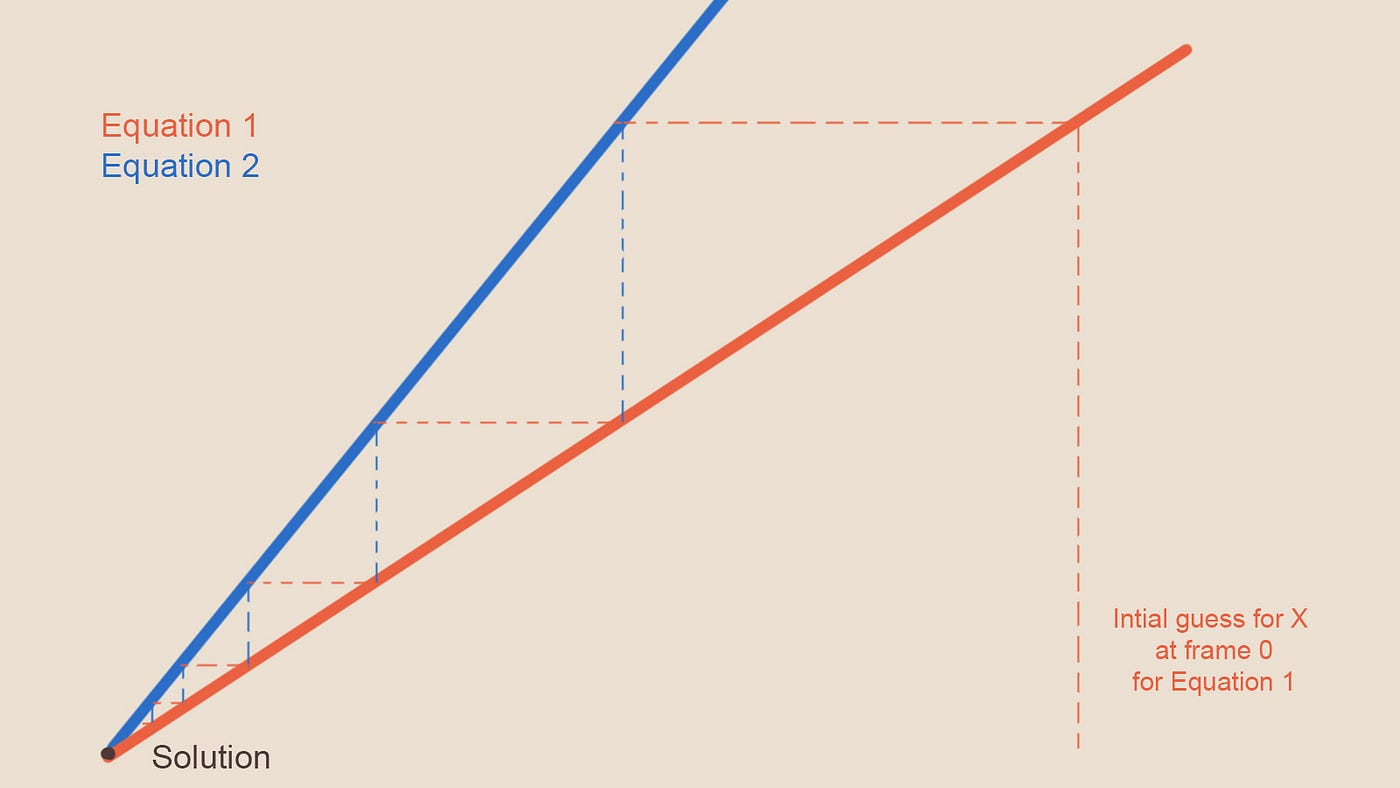Figure -13- Solvers in the Nth step, always coming closer to the answer but never reaching it

Of course this doesn’t work for all equations. Two graphs might not converge or have more than one solution etc. But in our case it does work.

To use our solver we rewrite the equation so that the field in the middle is on the left hand side, and for any given cell with coordinate i and j, we have:

`Solve(Velocity_Divergence){p_new[i, j] =((p[i+1,j] + p[i,j+1] + p[i-1,j] + p[i,j-1]) - Velocity_Divergence[i, j])/4}`

Once this runs we can do:

`CopyOldToNew(){p[i, j] = p_new[i, j]}`

And repeat the calculation again with the new values of p. We repeat this for around 30 steps until we have our solution for that frame. For the first step, we take p to be zero.

So put together our projection will look like this:

`Velocity_Div_field = Divergence(velocity_field); for(int i = 0; i < 30; i++) {   Solve(Velocity_Div_field);   CopyOldToNew();}deltaP = Gradient(p);new_divergent_free_velocity = velocity_field - deltaP;`

Of course the above is pseudo code, you wouldn't write your compute shader pipeline like that. But rather create buffers for all these, and set sources/ destination and call the kernels.

Notice that after calculating the pressure, I calculate the gradient of it, in order to pluck it in the equation we had, tying together the velocity field we started with, and the divergence free version we want to end up with.

This was all there is to projection. In the code, I have all the above wrapped around in a function called Project(), which you can call whenever you want.

# Diffusion Implementation

The first problem with compressibility is solved through projection, now we can address the instability of our diffusion calculation function which was introduced through it being formulated explicitly.

If you remember from above, given any field d, where d0 was the value of the field for each cell in the previous frame, our explicit formulation was:

`d_X= d0_X + diffusionFactor * deltaTime *(d0_01 + d0_02+ d0_03 + d0_04 -4*d0_X)`

In the diffusion case it is easy to see the source of instability, for large delta time or diffusion rates, if the amount of density coming from the neighboring cells is smaller than what is leaving from the central cell and the diffusionFactor*deltaTime is bigger than 1, then our density value for the new frame will become negative, which makes no sense.

We can formulate our diffusion differently, as an equation which when you diffuse the value of the field in the next frame backward in time, it give us the initial value we started with. So:

`d0_X = d_X - diffusionFactor * deltaTime * (d_01 + d_02+ d_03 + d_04 -4*d_X)`

Notice how we are not diffusing d0 but d, the value for the next frame. To understand the above equation intuitively, you need to understand what diffusion does. Diffusion looks at how the amount of something in the central cell compares to the average of its surrounding, if there is more in the center, it takes abit away from it, if the center has less than the average of its surrounding, it adds abit to it. This leads to the values balancing each other out over time and reach a uniform state. Since we assume as time goes forward things balance each other out, the opposite must be true if you look at the process backwards (move back through time). There the anti diffusion happens, where areas that have more density than the average of their surroundings, suck in more particles. This opposite of diffusion function is what the second part of our above equation is.

But why is this more stable or a better formulation? If you reformulate the above equation (which we need to do any ways) so that the new value of d for the central cell (X) is alone on the left hand side you will get this:

`d_X = (d0_X + diffusionFactor * deltaTime * (d_01 + d_02+ d_03 + d_04)) / (1 + 4 * diffusionFactor * deltaTime)`

Notice how in contrast to the explicit formulation, there is only addition here. So there is no chance our new density value is going to be negative, regardless of the size of delta time or diffusionFactor. So d_x will always remain positive. It is important to mention again that stability and accuracy are not the same. For a large delta time or diffusion rate, you won’t get meaningful numbers. At least it won’t blow up anymore!

You might have noticed at this point that the above formulation is very similar to the one we had for projection. Instead of d_X, there we calculated pressure in the center cell. d_01 to d_04 were the neighbouring pressure values and d0_x was divergence(velocity_field).

Just like projection, this seems impossible to solve at first glance (one equation and 5 unknowns, d_x and d_01 to d_04), but this is also a system of linear equations. One equation for each cell, N*N in total. The good news is, we can solve it with the solver we already have.

You can either write a different kernel/ shader for the solver of projection and diffusion, or reformulate the equations for both in a more general way so that by setting a certain parameter, you could use the same code for both equations, which is what I ended up doing in code: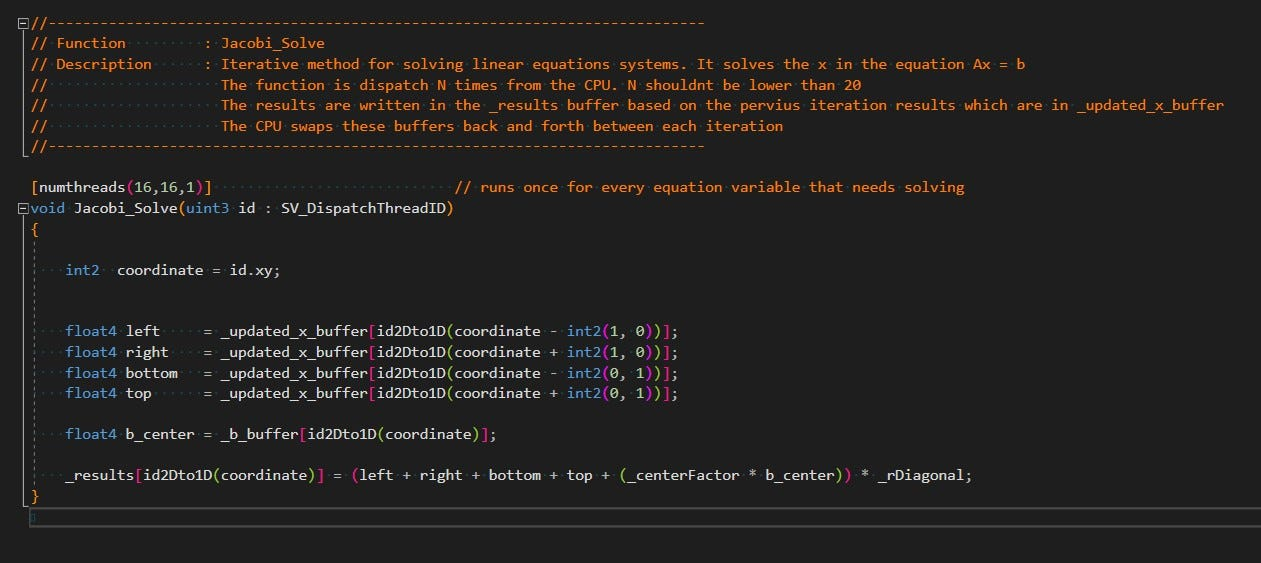By changing _rDiagonal and _centerFactor and switching _b_buffer to be divergnece(velocity) in one and d_x0 in the other, I can use the same solver for both equations

Last but not least, we need to fix our advection so that it uses a gather operation instead of the scatter operation.

We can use one of our learnings from the diffusion implementation to do that. In diffusion we formulated our equation based on what needs to be true, in the case we diffuse back in time. Instead of looking a head in time (next frame) and ask ourselves how our velocity will advect (move) our different fields, we can look back and formulate what quantities our current velocity has carried over from other cells from the previous frame.

In this setup our advected values will always be a frame behind, which is not an issue for our simulation. Based on the diagram, to calculate the advected part for any given cell for this frame:

field_amount_new_frame[cellPosition] += field_amount_old_frame[cellPosion-current_cell_velocity * timestep ]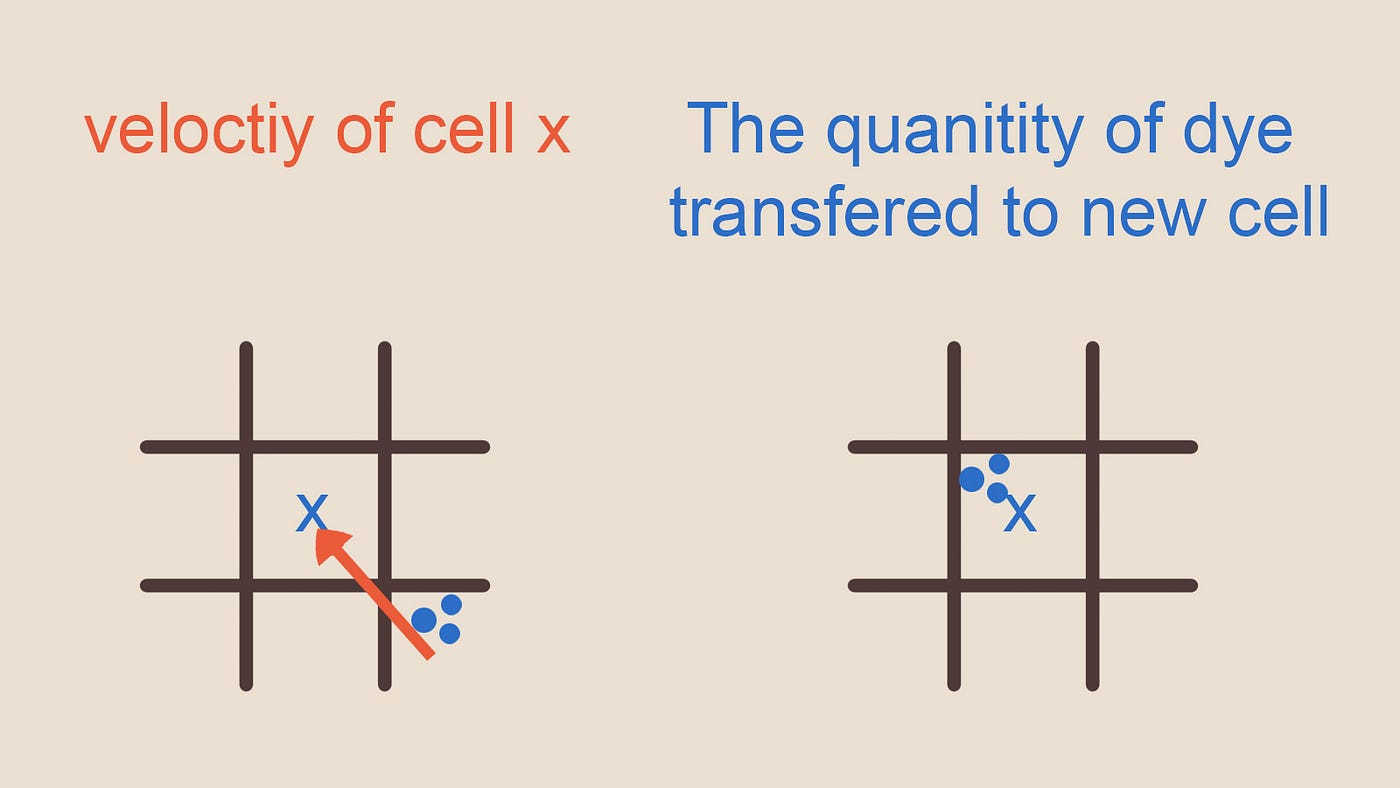Figure -14- Notice how this compares to the figure for a scattering version. quantities are always added to the cell that is being calculated and not carried off to some other location

# Some Last Points

That was it for the main fluid simulation. With this, the code (which is well documented) and the documentation on Github, you should be able to have a simulation running that you actually understand.

For a simple simulation, your setup would look like this (I do project several times, because it looks better that way):

There is a lot of stuff in the repo which I haven’t explained here, such as boundary handling, arbitrary boundary handling, fake 3D simulation, lighting, reflection, caustics etc. I will try to address each with a few lines here, since the article is already long as is.

## Boundaries and Arbitrary Boundaries

The operators we defined (divergence, gradient etc), will have a problem on boundary edges. Since the neighboring pixels are outside of the simulation grid. To solve this, we run a different program for the four corners of the grid.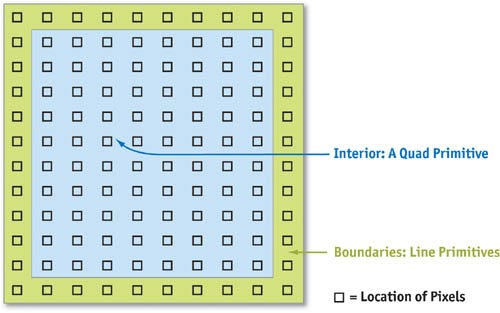Figure -15- GPU Gems chapter 38, the interior and boundaries cells

On the boundary cells, you can then do a very specific behavior depending on the field you are processing. For velocity you flip the velocity along the direction of the normal of the boundary, for pressure you set it the same as the neighboring cell so that Gradient(pressure) is zero, for density you can set it so that the boundary always have 0 density (up to you) etc. Below is the boundary handling in the compute shader.

Arbitrary boundaries are a bit more difficult to handle.

You would need A) a mask that determines where these boundaries are within your simulation grid, B) you would need a buffer containing the normals of these boundaries on those cells. You can hand paint the mask, or you can bake it in engine (using depth information of obstacles and the simulation grid, this allows for moving obstacles and rebaking every frame).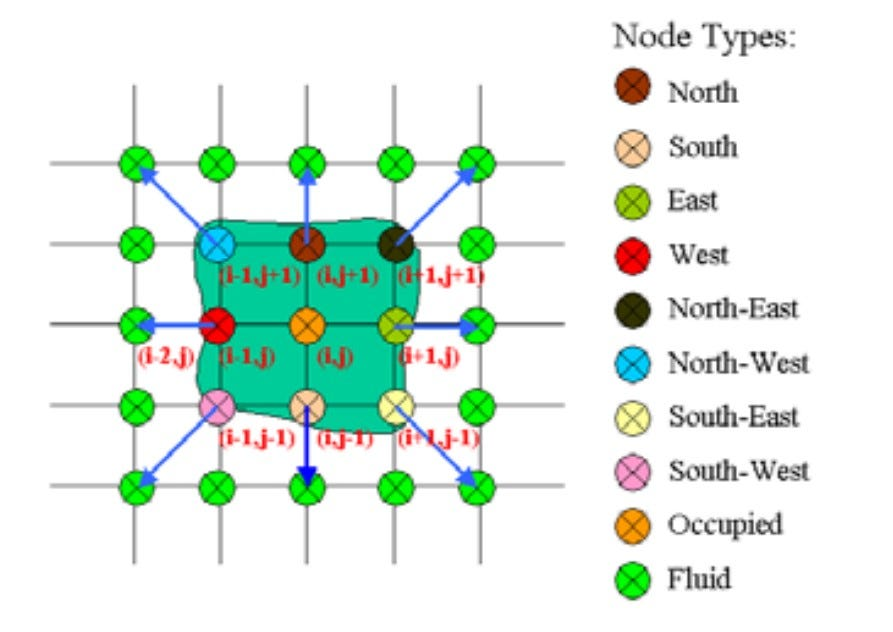Figure -16- Arbitrary normals boundaries diagram from An Improved Study of Real-TimeFluid Simulation on GPU, Enhua Wu et al.

I bake this map using compute shaders and an array containing all potential permutations of the above and their normals. The indices to this array are a bit mask of a sort, which I won’t get into, since it is an article of its own. The bitmask allows me to update the normals buffer very fast whenever the dynamic boundaries change, without branching in shader. Here is the code for creating the normals look up array in the CPU and using that to generate the boundaries normals buffer in compute shader and the code that runs once per cell to deal with the different fields of the fluid simulation which are within or bordering an arbitrary boundary.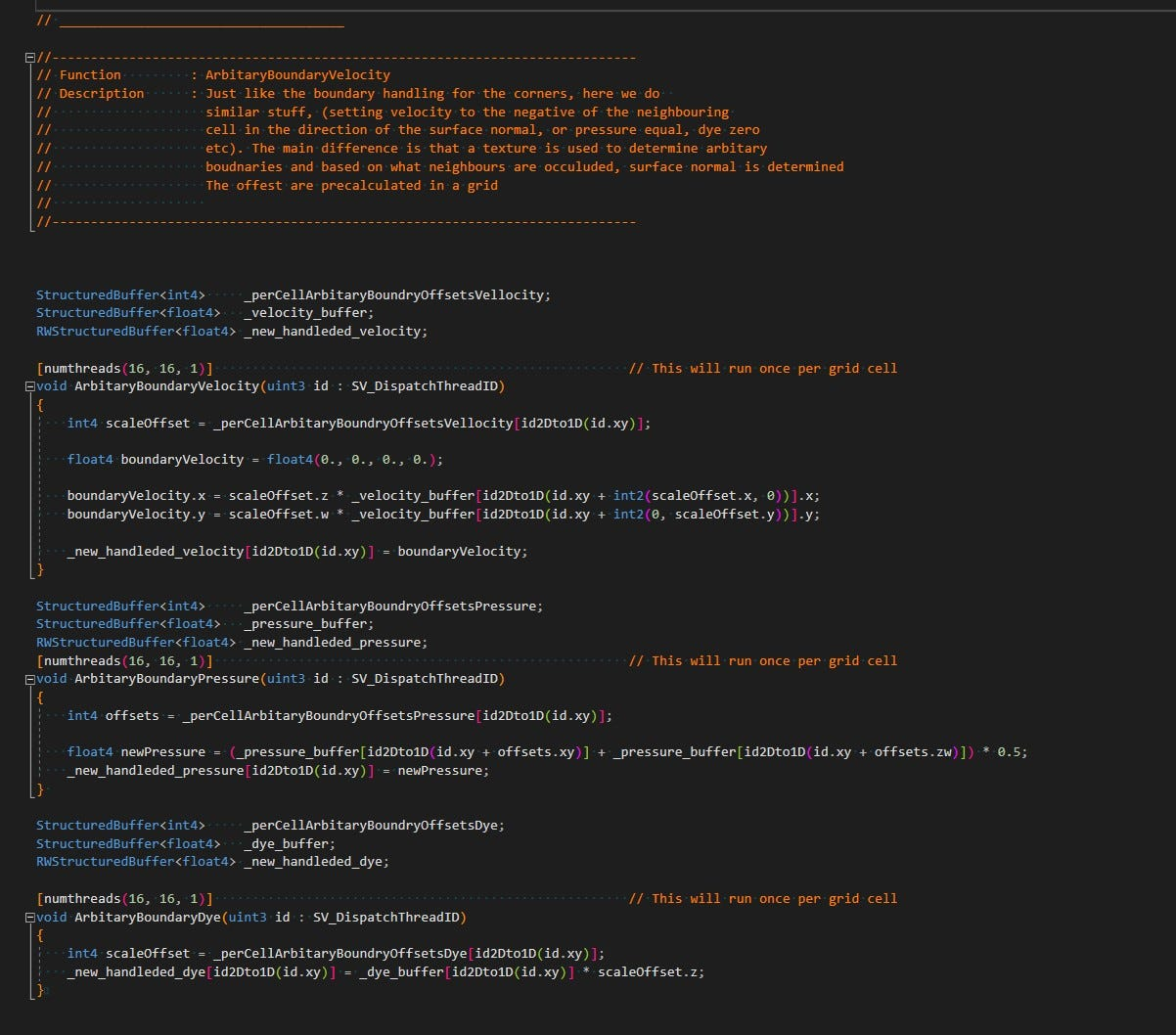Arbitrary boundaries, the code includes the baking of the look up table as well as the actual shaders

## Fake 3D Simulation

In my Persian Garden Demo Scene, you can see that the water simulation looks 3D. This is not a 3D simulation however and the underlying grid is still 2D. I am using the pressure buffer (tone mapped to a reasonable range), to determine whether I should push the vertices of the water surface up or down. This physically kind of makes sense, since if it was a 3D simulation, some of the water would move up when there is higher pressure difference and less to the sides. However it is a hack, a good looking one though, at way less cost compared to doing an actual 3D water simulation.

## Lighting

I have costume lighting on all the objects in the demo scene. It is a variation of the standard Cook-Torrance BRDF, which you can read more about here. The water itself consists of:

1. Specular lighting from directional light
2. Refraction (for which I am simply garbing the texture behind the water and showing it with distortion/ displacement based on the movement on the surface of the water)
3. Reflection

Here for example you can see the specular part of the lighting from the directional light

I also have a bit of diffuse lighting in there, for the case the water is muddy. However this effect is not finished, as it still requires SSS and volumetric shadows/ light shafts. A project for another time :)

## Reflection

The reflection in the demo has two parts. First part is a planar reflection:

However the planar reflection alone is not enough for cases where there is extreme displacement. As showcased in the diagram below, it works well in case 1, but for the edge cases I needed to combine it with screen space reflection, hence the solution has both.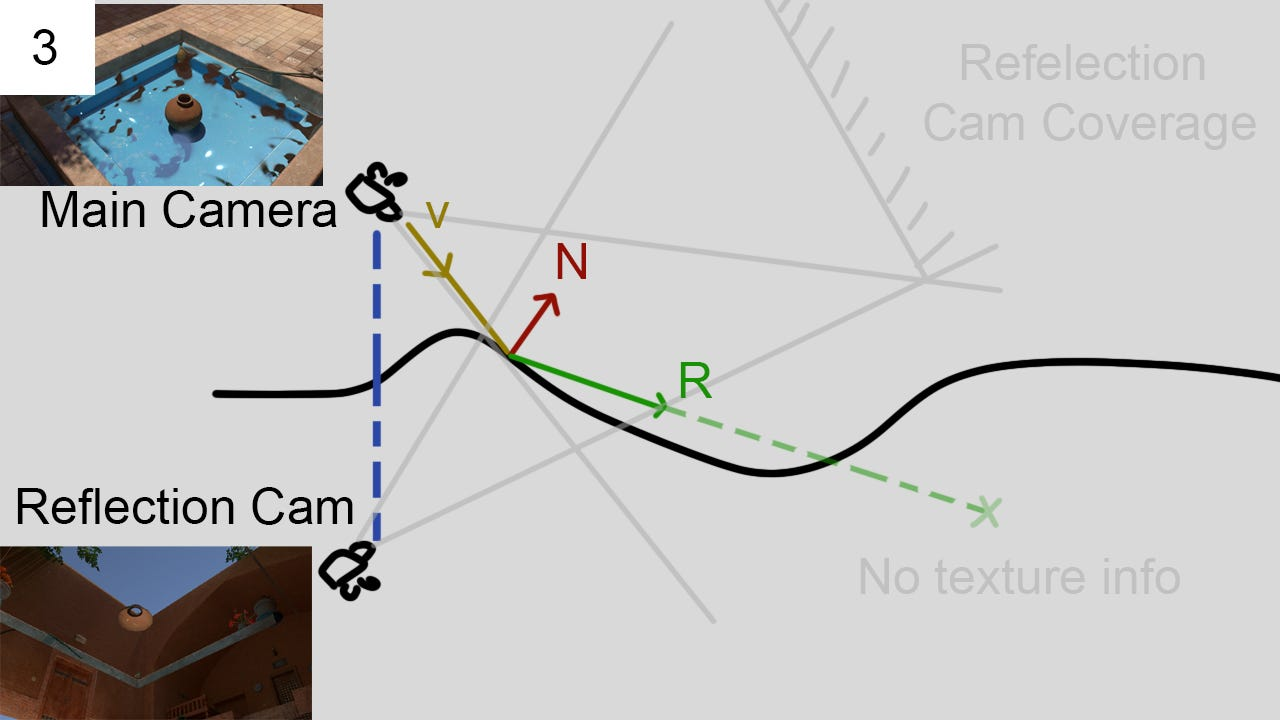Figure -18- The planar reflection alone is not enough for extreme displacement

## Caustics

My approach to caustics is pretty standard. There are two type of caustics: 1) under water, 2) reflected out of the water.

Both work with projection. I project the pressure texture from the light position on to the ground, and take the first derivative (sobel filter) of the tone mapped pressure buffer to be the sources of my caustics. Physically this is the opposite of where the caustics should pop up, but since it looked better, I went with this. To enhance the wavelength depended refraction look, the applied caustics on the ground surface has different offsets to how the pressure buffer is read for its RGB channels, causing a slight rainbow like soft edge on the caustics.

The second type is above the water. Also a projection, but I simply change the projection direction based on which side of the fountain is being rendered (the permutations are pre baked in a 4x4 matrix, similar to arbitrary boundary).

## Fish Animation

The fish reacts to your activity in water. Their animation is fully procedural, through overlapping sinus waves along the different axis, and masking through the vertex positions in object space.

## Micro Details

Your fluid simulation can only be so dense. Even with more optimized solution there is a limit to the amount of detail you can add through it. Hence, it might be a good idea to add some visual noise to your simulation, to fake a higher density simulation. I only dabbled with this at the very end, but didn’t dive in. The main challenge is to make sure the noise looks like it belong to the fluid.

## Further Development?

If you read this and liked it, where do you go to read more on it? Resources mentioned below are a good one, on top of that Stam (author of fluid dynamic for games) has a book where he goes into more details. There is also a lot you can add to where I left the implementation. Your biggest gain would probably be in upgrading the solver to a multi grid. Further optimization could be using textures instead of structured buffers (to use texture caches), or the use of group shared memory for the solver. Also the fluid engine was written for explanatory proposes. At times I use larger textures as I need, or buffers with higher precision as needed. Some buffers are doubled in memory for ease of use, and generally the whole pipeline can be optimized further. Another area where further research and fine tuning is worth it, is looking into better tone mapping algorithm to convert the pressure buffer into a displacement map, as well as combing traditional “fake” water flow techniques with the simulation to add micro details.

Thanks for reading and I hope the article helped to demystify fluid simulation or even a whole family of iterative methods commonly used in engineering. For more, you can follow me on Twitter: IRCSS

# Resources

1. Stam 1999 Paper on stable fluid Dynamic, this is pretty much the god father of this method in compute graphics: https://www.researchgate.net/publication/2486965_Stable_Fluids
3. Harris, Fast Fluid Dynamics Simulation 2004 in GPU gems 3, chapter 38, it is the adoptation of the Stam 1999 paper with a Jacobi solver on the GPU: https://developer.download.nvidia.com/books/HTML/gpugems/gpugems_ch38.html
4. Stam 2003 Real Time Fluid Dynamics for Games. Not sure what the difference between this one and 1999 paper is beside the solver being a Gauss Seidel relaxation solver. The 2003 kind of glosses over the projection method, but seems to use the same Poisson equation with pressure: https://www.researchgate.net/publication/2560062_Real-Time_Fluid_Dynamics_for_Games
5. Adoption of the Stam 2003 for 3D and exploring rendering methods by Mike Ash: https://mikeash.com/pyblog/fluid-simulation-for-dummies.html
6. Amador et al 2012, Linear Solvers for Stable Fluids: GPU vs CPU, discussion around the linear solvers available for GPU architecture: https://www.it.ubi.pt/17epcg/Actas/artigos/17epcg_submission_39.pdf
7. Fedkiw et al. 2001 Visual Simulation of Smoke, method of bringing back vortices through vortices confinement which are lost in the simulation: https://www.researchgate.net/publication/2390581_Visual_Simulation_of_Smoke
8. Ďurikovič 2017, Real-Time Watercolor Simulation with Fluid Vorticity Within Brush Stroke: https://www.researchgate.net/publication/321112340_Real-Time_Watercolor_Simulation_with_Fluid_Vorticity_Within_Brush_Stroke
9. Curtis et al. 1997 Computer-Generated Watercolor: https://www.researchgate.net/publication/2917328_Computer-Generated_Watercolor
10. Enhua Wu et al. 2004, An Improved Study of Real-Time Fluid Simulation on GPU: http://citeseerx.ist.psu.edu/viewdoc/download?doi=10.1.1.99.7898&rep=rep1&type=pdf
12. Rendering water as post process: https://www.gamedev.net/articles/programming/graphics/rendering-water-as-a-post-process-effect-r2642/
13. Resource used for lighting the water, learn open gl: https://learnopengl.com/PBR/Lighting
14. Catlike coding on water shading: https://catlikecoding.com/unity/tutorials/flow/looking-through-water/
15. Realtime realsitic occean lighting https://hal.inria.fr/inria-00443630/file/article-1.pdf
16. Wikipedia article on explicit and implicit numeric methods and stability: https://en.wikipedia.org/wiki/Explicit_and_implicit_methods
17. Arbitrary boundaries, An Improved Study of Real-Time

--

--

--

## More from Shahriar Shahrabi

Technical Artist

Love podcasts or audiobooks? Learn on the go with our new app.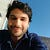Technical Artist

## Realtime Procedural Audio and Synthesized Piano in Unity 3D## Unity : AI && NavMesh [FSM : (Finite State Machine with Enum)]## All about Sky in Unity’s HDRP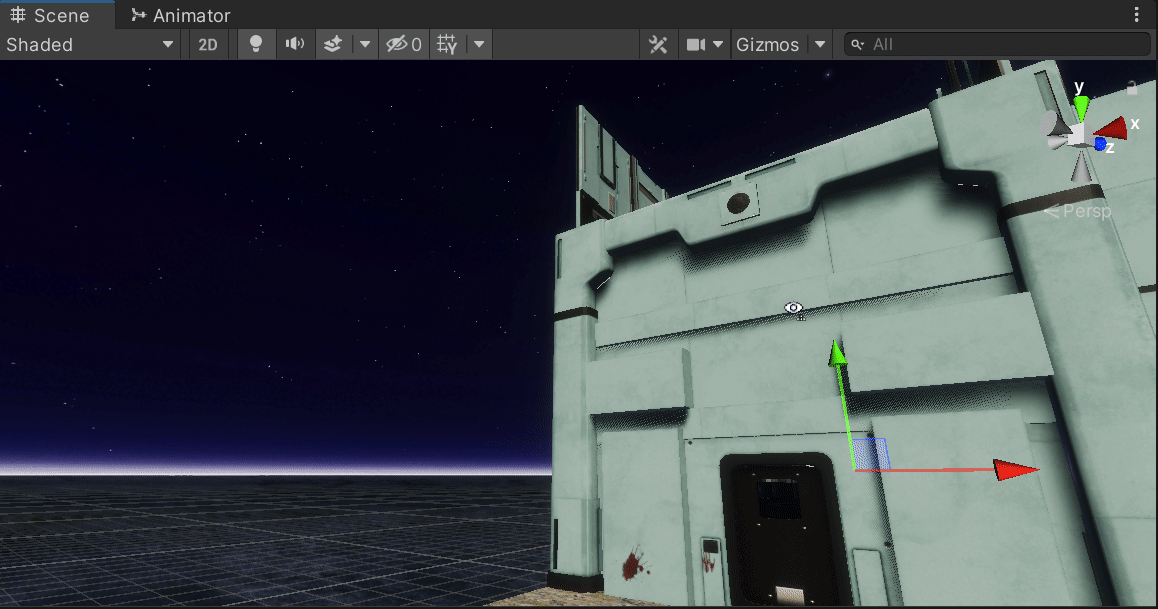## So, you want to use multithreading in Unity WebGL